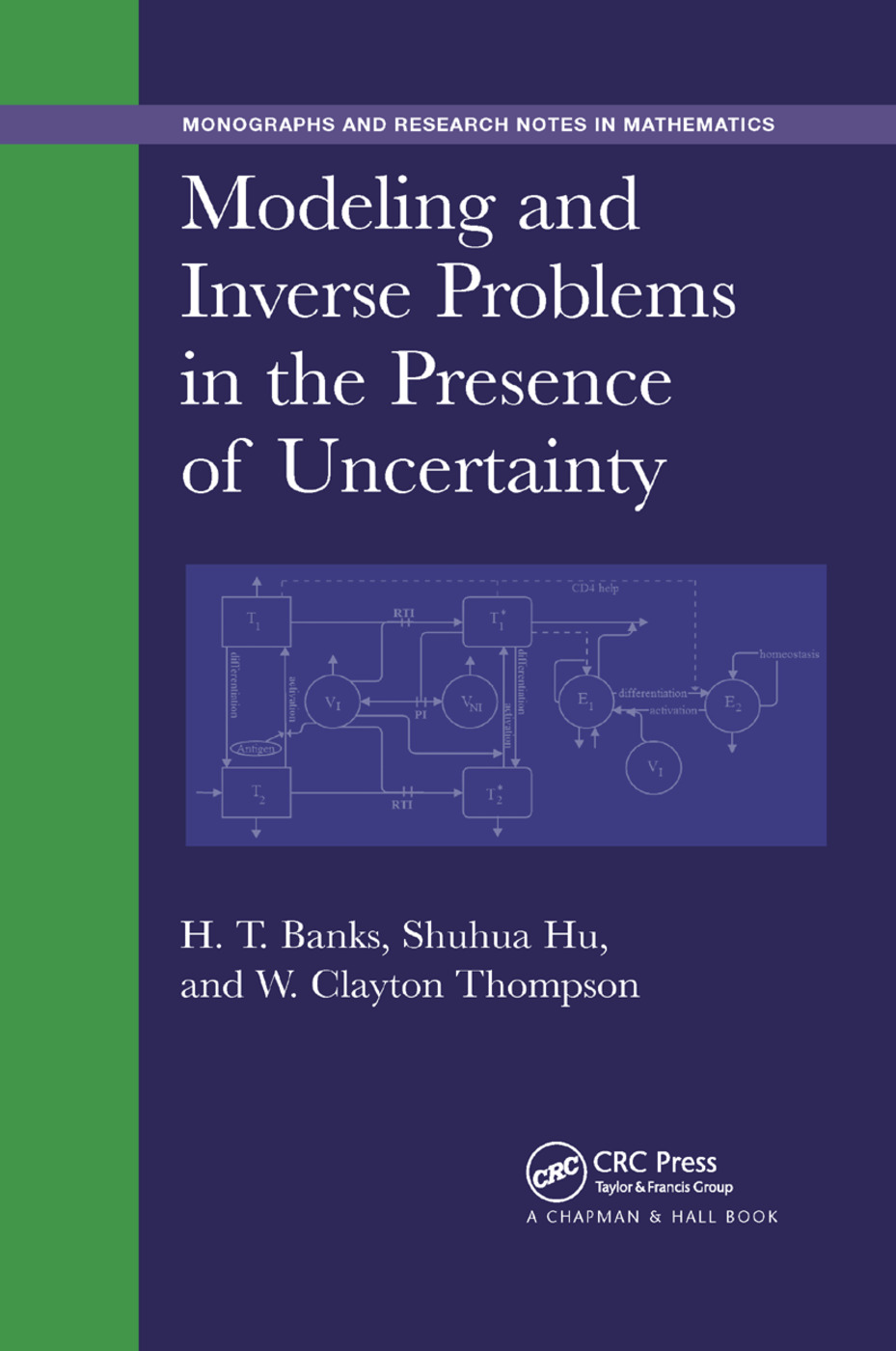# Modeling and Inverse Problems in the Presence of Uncertainty

## 1st Edition

Chapman and Hall/CRC

405 pages

Paperback: 9780367378752
pub: 2019-09-19
SAVE ~\$14.99
\$74.95
\$59.96
x
Hardback: 9781482206425
pub: 2014-04-01
SAVE ~\$25.00
\$125.00
\$100.00
x
eBook (VitalSource) : 9780429171024
pub: 2014-04-01
from \$28.98

FREE Standard Shipping!

### Description

Modeling and Inverse Problems in the Presence of Uncertainty collects recent research—including the authors’ own substantial projects—on uncertainty propagation and quantification. It covers two sources of uncertainty: where uncertainty is present primarily due to measurement errors and where uncertainty is present due to the modeling formulation itself.

After a useful review of relevant probability and statistical concepts, the book summarizes mathematical and statistical aspects of inverse problem methodology, including ordinary, weighted, and generalized least-squares formulations. It then discusses asymptotic theories, bootstrapping, and issues related to the evaluation of correctness of assumed form of statistical models.

The authors go on to present methods for evaluating and comparing the validity of appropriateness of a collection of models for describing a given data set, including statistically based model selection and comparison techniques. They also explore recent results on the estimation of probability distributions when they are embedded in complex mathematical models and only aggregate (not individual) data are available. In addition, they briefly discuss the optimal design of experiments in support of inverse problems for given models.

The book concludes with a focus on uncertainty in model formulation itself, covering the general relationship of differential equations driven by white noise and the ones driven by colored noise in terms of their resulting probability density functions. It also deals with questions related to the appropriateness of discrete versus continuum models in transitions from small to large numbers of individuals.

With many examples throughout addressing problems in physics, biology, and other areas, this book is intended for applied mathematicians interested in deterministic and/or stochastic models and their interactions. It is also s

Introduction. Probability and Statistics Overview. Mathematical and Statistical Aspects of Inverse Problems. Model Comparison Criteria. Estimation of Probability Measures Using Aggregate Population Data. Optimal Design. Propagation of Uncertainty in a Continuous Time Dynamical System. A Stochastic System and Its Corresponding Deterministic System. Frequently Used Notations and Abbreviations. Index.

Banks, H. T.; Hu, Shuhua; Thompson, W. Clayton